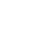# Use RxJS mapTo and map to Transform Values Emitted by Observables

Published 6 years ago
Updated 8 months ago

transforming the values emitted by observables. We made our first operator called multiplyBy, which looks a bit useful, but in practice we don't need it because it's too specific: it only does simple multiplication on numbers. In this lesson we will see how the map() operator is useful for any calculation on delivered values.

🚨 Since we are importing `interval` from RxJS, we don't need to preface our Observables with `Rx.Observable`. You can no longer `.{operator}`, you need to `.pipe({operator})` instead.

[00:00] We made our first operator called multiplyby which looks a bit useful, but in practice we don't need it because it's too specific, it only does simple multiplication on numbers. What if we wanted to divide by 10, or let's say divide and subtract? Or even more general, calculate the result of quite a large formula. It's clear that it's not a good idea to have an operator for each of these specific cases, we need an operator that simply does calculations.

[00:26] Let's call that one calculate for now, and let's rename them down here. Calculate instead of taking a multiplier which just a number, it will take a transformation function. Inside the transformation function is where we can say are we going to multiply, are we going to divide, or subtract, and etc. Instead of a number we can give a function such as an arrow function, which takes x and will return x * 2.

[00:55] X is the value emitted on the source observable, which is foo for instance, and the x * 2 is the value delivered on the result observable which is bar. The transformation function we need to use it here instead of multiplier. We apply that transformation function on the x, which is the value that the source just emitted. This function will return us a value just like it's returning here, x * 2, and that's what we're going to deliver on observer.next for the result observable.

[01:31] So if we run this, we see now next 02, 4, just like we wanted, and instead of multiplying we could also say, hey, I want to divide, and then we should see 0and then half, and then 1, etc. In RSJX we have this function already provided to us and it's called actually map. So I'm going to delete this because we don't need that anymore, and our calculate is actually called map.

[02:06] Map is a really useful operator, it's quite common to see it. As we can see it still works, and the idea and why is it called map is that when you think of a map in real life, it is representing an area like, let's say, a geographical area onto paper. So you're moving each of these values onto this other space, so we're just mapping each of these values using this transformation here. In case you have map function which will always return the same value, let's say given x we're always going to return 10.

[02:48] So if you run that, we just deliver 10 every time. If that's your case, then we're actually transforming this x, we're actually just dropping on the floor and using 10 anyway. Another way of writing this in ES6 would be like this, so empty parenthesis and 10, but in RSJX we have a small optimization which is in those cases where you just have a constant you can say mapto to this constant 10, and that does the same thing.

[03:23] The big lesson for learning map is that it exists as the most basic way of transforming the values emitted by observables.Vance
~ 4 years ago

would we actually monkey patch Observable with our own methods in reality? I'm guessing this is just rhetorical/socratic.